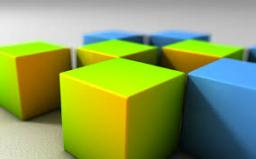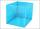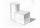# Cube edge

Determine the edges of the cube when the surface is equal to 37.5 cm square.

a =  2.5 cm

### Step-by-step explanation:Did you find an error or inaccuracy? Feel free to write us. Thank you!## Related math problems and questions:

• Cube edgesThe sum of the lengths of the cube edges is 42 cm. Calculate the surface of the cube.
• Cube 5The content area of one cube wall is 32 square centimeters. Determine the length of its edges, its surface and volume.
• The cubeThe cube has a surface of 600 cm2. What is its volume?
• Cube 5The surface of the cube is 15.36 dm2. How will change the surface area of this cube if the length of the edges is reduced by 2 cm?
• Sum of the edgesThe sum of the lengths of all edges of the cube is 72 cm. How many cm2 of colored paper are we going to use for sticking?
• Wallpaper3750 cm square of wallpaper is needed to glue a cube-shaped box. Can Dad cut out the whole necessary piece of wallpaper as a whole if he has a roll of wallpaper 50 cm wide?
• Cuboid surfaceDetermine surface area of cuboid if its volume is 52.8 cm cubic and length of the two edges are 2 cm and 6 cm.
• Cube V2SThe volume of the cube is 27 dm cubic. Calculate the surface of the cube.
• The cubeThe cube has a surface area of 486 m ^ 2. Calculate its volume.
• Cuboid to cubeA cuboid with dimensions of 9 cm, 6 cm, and 4 cm has the same volume as a cube. Calculate the surface of this cube.
• A butterA butter cube with an edge 6.5 cm long is packed in a package with dimensions a = 28 cm, b = 15 cm. Calculate how many cm2 the package is larger than the surface of the cube.
• CubeThe sum of all cube edges is 30cm. Find the surface area of the cube.
• CubeHow many times increases the surface area of a cube with an edge 23.4 cm if the length of the edge doubles?
• Surface area of cylinderDetermine the lateral surface of the rotary cylinder which is circumscribed cube with edge length 5 cm.
• Edges or sidesCalculate the cube volume, if the sum of the lengths of all sides is 276 cm.
• Edge cFind the edge c of cuboid if an edge a = 20 mm, b = 30 mm and surface area S = 8000 mm2.
• Surface of cuboidFind the surface of the cuboid if its volume is 52.8 cm3 and the length of its two edges is 2 cm and 6 cm.